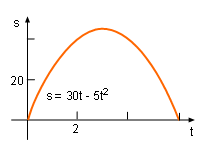Ch 4. The Mean Value Theorem Multimedia Engineering Math Maximum & Minimum Rolle'sTheorem Mean ValueTheorem MonotonicFunctions First DerivativeTest Concavity &Inflection SecondDerivative Test
 Chapter 1. Limits 2. Derivatives I 3. Derivatives II 4. Mean Value 5. Curve Sketching 6. Integrals 7. Inverse Functions 8. Integration Tech. 9. Integrate App. 10. Parametric Eqs. 11. Polar Coord. 12. Series Appendix Basic Math Units Search eBooks Dynamics Fluids Math Mechanics Statics Thermodynamics Author(s): Hengzhong Wen Chean Chin Ngo Meirong Huang Kurt Gramoll ©Kurt GramollMATHEMATICS - CASE STUDY IntroductionKicking Ball A ball is kicked upward and then falls back down to its initial spot. Eddy wonders the maximum altitude will be if he knows the altitute as a function of time. . What is known: The altitude function of the kicked ball is s = 30t - 5t2. The units for displacement and velocity are m and m/s respectively QuestionsDisplacement Diagram What is the maximum altitude the ball goes up? Approach At the maximum altitude, the velocity of the ball is 0. Recall that velocity is simply the time derivate of displacement.